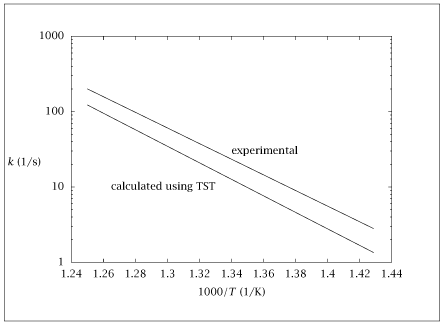## Figure 5.5:

### Comparison of measured and calculated rate constant versus temperature for trioxane decomposition.## Code for Figure 5.5

### main.m

```
1
2
3
4
5
6
7
8
9
10
11
12
13
14
15
16
17
18
19
20
21
22
23
24
25
26
27
28
29
30
31
32
33
34
35
36
37
38
39
40
41
42
43
44
45
46
47
48
49
50
51
52
53
54
55
56
57
58
59
60
61
62
63
64
65
66
67
68
69
70
71
72% Copyright (C) 2001, James B. Rawlings and John G. Ekerdt
%
% This program is free software; you can redistribute it and/or
% modify it under the terms of the GNU General Public License as
% published by the Free Software Foundation; either version 2, or (at
% your option) any later version.
%
% This program is distributed in the hope that it will be useful, but
% WITHOUT ANY WARRANTY; without even the implied warranty of
% MERCHANTABILITY or FITNESS FOR A PARTICULAR PURPOSE.  See the GNU
% General Public License for more details.
%
% You should have received a copy of the GNU General Public License
% along with this program; see the file COPYING.  If not, write to
% the Free Software Foundation, 59 Temple Place - Suite 330, Boston,
% MA 02111-1307, USA.
%
% Revised 7/24/2018

%  na = 6.02252e23;
%  mo = 15.9994;
%  mh = 1.0079;
%  mc = 12.011;
R1 = 1.987; % Gas Constant (cal/mol/K)
R = 8.314; % Gas Constant (J/mol/K)
%  Rg = 82.06; % Gas Constant (cm^3*atm/K/mol)
kb = 1.381e-23; % Boltzmann Constant (J/K)

T = linspace(700,800,11); % Temp (K)
%  T = 750; % Temp (K)
h = 6.626e-34; % Planks Constant (J*s)
c = 2.998e10; % Speed of Light (cm/s)

nucompl = [100, 100, 200, 200, 945, 945, 1400, 1400, ...
1178, 1178, 1200, 1200, 1200, 1200, 1481, ...
1481, 2850, 2850, 3025, 3025, 1000, 1242, ...
1100, 200, 700, 1200, 1495, 2850, 3025]';
%frequencies of transition complexes (1/cm)
nuoxir = [296, 296, 524, 524, 945, 945, 1070, 1070, ...
1178, 1178, 1305, 1305, 1410, 1410, 1481, ...
1481, 2850, 2850, 3025, 3025, 1122, 1242, ...
1383, 466, 752, 978, 1235, 1495, 2850, 3025]';
%frequencies of trioxane (1/cm)

E = 51400; %potential energy barrier (cal/mol)
IaIbIc_compl = 125.3*120.5*249.2; %moments of inertia of transition complexes (amu-A^2)
IaIbIc_oxir  = 96.4*96.4*173; %moments of inertia of trioxane (amu-A^2)
qrot_ratio = ((IaIbIc_compl)^0.5)/((IaIbIc_oxir)^0.5);

freq       = (kb)*T/h; % (1/s)
hc         = h*c ./ (kb*T); %(cm)
qvibcompl  = exp(-0.5*nucompl*hc) ./ (1-exp(-nucompl*hc));
qviboxir   = exp(-0.5*nuoxir*hc) ./ (1-exp(-nuoxir*hc));

prodcompl  = prod(qvibcompl);
prodoxir   = prod(qviboxir);
qvib = prod(qvibcompl) ./ prod(qviboxir);

qe   = exp(-E./(R1*T));
k    = freq.*qrot_ratio.*qe.*qvib; % (1/s)
kexp = (10^15.28)*exp(-47500./(R1*T));
kup  = (10^(15.28+0.06))*exp(-(47500-2.4)./(R1*T));
klow = (10^(15.28-0.06))*exp(-(47500+2.4)./(R1*T));

table = [1000./T', k', kexp', kup', klow'];
save -ascii trioxane.dat table;

if (~ strcmp (getenv ('OMIT_PLOTS'), 'true')) % PLOTTING
semilogy (table(:,1),table(:,2:3));
axis([1.24 1.44 1E0 1E3])
% TITLE
end % PLOTTING

```